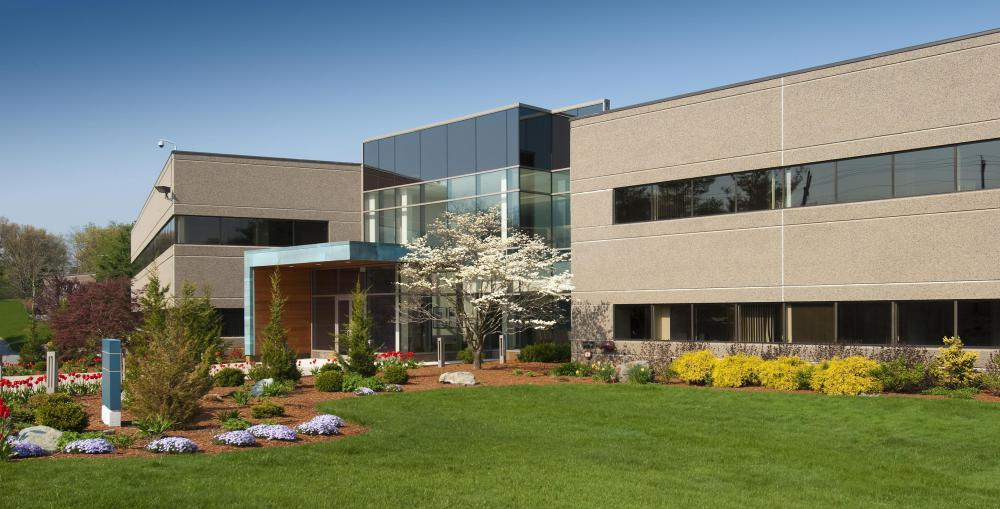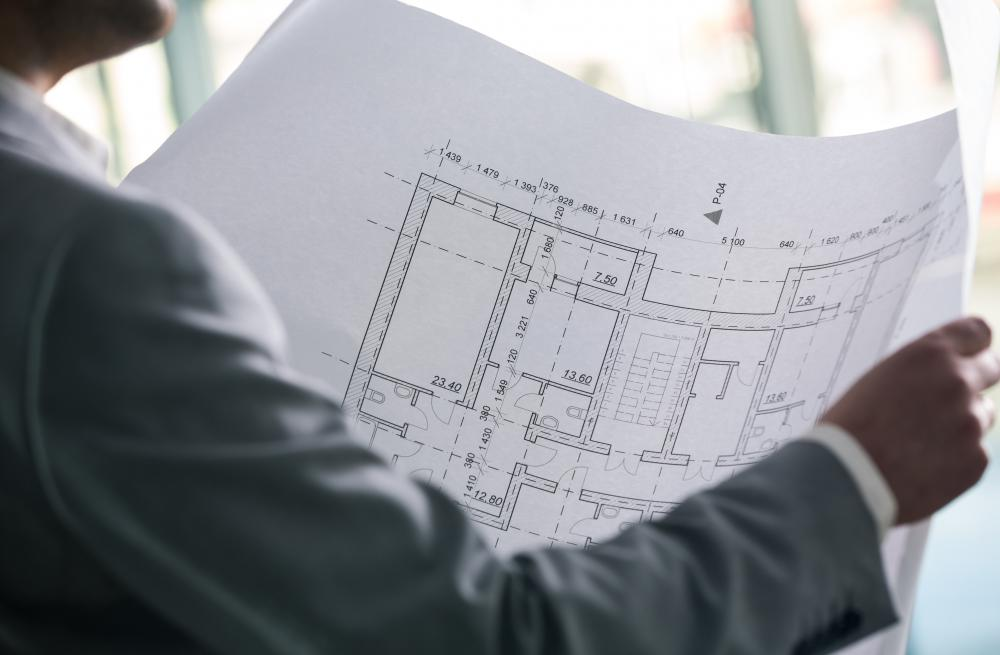# How do I Calculate Building Depreciation?

Patrick Lynch

Depreciation can be defined as the amount an asset or building loses in value over the course of time due to inevitable factors such as deterioration. Building depreciation can be calculated in a variety of ways including straight-line depreciation or via the reducing balance method. Straight-line building depreciation is calculated by estimating the value of the property at the end of its useful life and using its current value to subtract a set percentage from its worth each year. When the reducing balance method is used, a higher initial depreciation charge may be preferred but the subsequent losses become smaller each year.Reducing balance building depreciation ensures that a company does not overvalue its assets.

Straight-line building depreciation is used most frequently as it is the least confusing method of calculating the loss in value of property. It may not be completely accurate, but it does give the company’s bookkeepers a tangible figure to enter on the income statement which helps simplify the annual accounts. It is not easy to determine how much a building will depreciate over a long period of time; each property is different, so the straight-line method may not the best means of calculating the loss of such an asset.Building depreciation can be determined as early as the planning stages.

Suppose a company has a building currently valued at \$300,000 U.S. Dollars (USD) and decides the salvage value after five years will be \$150,000. It will subtract the final value from the current cost and divide it by the number of years of useful life. In this instance, \$300,000 - \$150,000/5 = \$30,000 annual depreciation. So at the end of year one, the building will be worth \$300,000 - \$30,000 = \$270,000. In its final year, the building will start at a worth of \$180,000 and end with at \$150,000.

Reducing balance building depreciation is more complex but gives a better idea of a property’s true worth. This is calculated by multiplying the depreciation rate by the current value of the property. The rate is usually determined by doubling the estimated straight-line percentage.

In the above example, the rate is 10% and the fixed value of the building is \$300,000. Using reducing balance building depreciation, the rate would be doubled to 20%. This means the first year’s depreciation is 20% of \$300,000 or \$60,000, thereby leaving the building’s value at the end of year one at \$240,000. Instead of using \$300,000 as the figure for the second year, a starting value of \$240,000 is used.

At the end of year two, the building would be worth \$192,000 or \$240,000 x 20%. The value of the property at the end of year five would be \$93,804. While this may not be anywhere near as high as the straight-line building depreciation method, it does ensure that a company does not overvalue its assets. In the world of business, it is usually better to underestimate the value of assets to avoid unpleasant surprises later.

## You might also LikeTalentryto

When it comes to the depreciation of a building, it is also important to think about repairs and updates that have been done as these factors may increase its value. In addition, buildings in some areas where real estate is at a premium are likely to increase in value because the location is considered to be in demand.